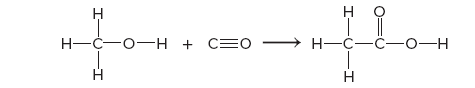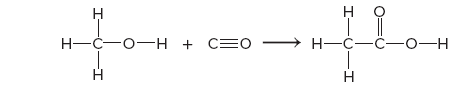# Problem: An important industrial route to extremely pure acetic acid is the reaction of methanol with carbon monoxide:Use bond energies to calculate the enthalpy of reaction.

###### FREE Expert Solution

Bond energies:Bond Energy (kJ/mol) # of Bonds (mol) Reactants Products C - H 413 1 x 3 = 3 1 x 3 = 3 O - H 463 1 x 1 = 1 1 x 1 = 1 C = O 799 0 1 x 1 = 1 C ≡ O 1072 1 x 1 = 0 0###### Problem Details

An important industrial route to extremely pure acetic acid is the reaction of methanol with carbon monoxide:

Use bond energies to calculate the enthalpy of reaction.